# The Azimuth Project Blog - network theory (part 19) (Rev #11, changes)

Showing changes from revision #10 to #11: Added | Removed | Changed

This page is a blog article in progress, written by John Baez and Jacob Biamonte. To see discussions of this article while it was being written, visit the Azimuth Forum.

joint with Jacob Biamonte

It’s time to resume the network theory series! It’s been a long time, so we’ll have assume to remind you of forgot some everything things. we’ve said before, and make this post as self-contained as possible.Last time we started looking at a simple example: a diatomic gas.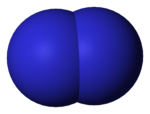Two atoms can recombine to form a diatomic molecule:

$A + A \to A_2$

and conversely, a diatomic molecule can break apart into two atoms:

$A_2 \to A + A$

We can draw both these reactions using a Petri net: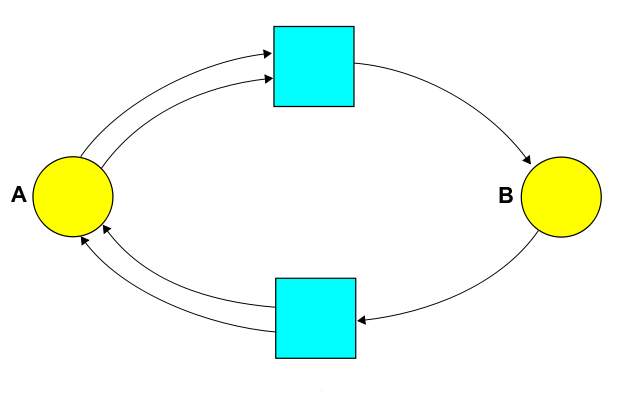where we’re writing $B$ instead of $A_2$ to abstract away some detail that’s just distracting here. Or, equivalently, we can use a chemical reaction network: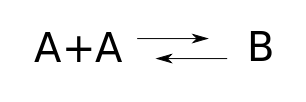Last time we looked at the rate equation for this chemical reaction network, and found equilibrium solutions of that equation. Now let’s look at the master equation, and find equilibrium solutions of that. This will serve as a review of three big theorems.

### The master equation

We’ll start from scratch, in case you’re just tuning in. The master equation is all about how atoms or molecules or rabbits or wolves or other things interact randomly and turn into other things. So, let’s write write $\psi_{m,n}$ for the probability that we have $m$ atoms of $A$ and $n$ molecule of $B$ in our container. These probabilities are functions of time, and master equation will say how they change.

First we need to pick a rate constant for each reaction. Let’s say the rate constant for this reaction is some number $\alpha > 0$:

$A + A \to B$

while the rate constant for this reaction is some number $\beta > 0$:

$B \to A + A$

Then Before we make it pretty using the ideas we’ve been explaining, the master equation says

 \begin{array}{ccl} \displaystyle{ \frac{d}{d t} \psi_{m,n} (t)} &=& = \alpha (m+2)(m+1)\psi_{m+2,n-1}(t) - \alpha m(m-1) \psi_{m,n}(t) + \\ && \beta (n+1) \psi_{m-2,n+1}(t) - \beta n \psi_{m,n}(t) \end{array}

Yuck! where we define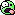$\psi_{i,j}$ Normally we don’t show you such nasty equations. Indeed the whole point of this series is to show be that zero by if packaging either the equations in a better way, we can understand them using high-level concepts instead of mucking around with millions of scribbled symbols. But we thought we’d show you the engine under the hood, just once.$i$ or $j$ is negative.

Each Yuck! term Normally we don’t show you such nasty equations. Indeed the whole point of our work has been to show you that by packaging the equations in a meaning. better For way, example, we can understand them using high-level concepts instead of mucking around with millions of scribbled symbols. But we thought we’d show you what’s secretly lying behind our beautiful abstract formalism, just once.

Each term has a meaning. For example, the first one:

$\alpha (m+2)(m+1)\psi_{m+2,n-1}(t)$

means that the reaction $A + A \to B$ will tend to increase the probability of there being $m$ atoms of$A$ atoms and$n$ molecules of$B$ molecules if we start with 2 more$A$‘s and 1 fewer $B$ ’s. . This reaction can happen in$(m+2)(m+1)$ ways if we start with $m+2$ atoms of $A$. And it happens at a probabilistic rate proportional to the rate constant for this reaction, $\alpha$.

We won’t go through the rest of the terms. It’s a good exercise to do so, but there could easily be a typo in the formula, since it’s so long and messy. So let us know if you find one!

To simplify this mess, the key trick is to introduce a generating function that summarizes all the probabilities in a single power series:

 \Psi = \sum_{m,n \ge 0} \psi_{m,n} z_1^m y^m \, z_2^n z^n

It’s a power series in two variables,  z_1 y and  z_2 z, since we have two chemical species: $A$ ‘s, ‘s and$B$’s.

Using this trick, the master equation looks like

$\frac{d}{d t} \Psi(t) = H \Psi(t)$

where the Hamiltonian $H$ is a sum of terms, one for each reaction. This Hamiltonian is built from operators that annihilate and create $A$‘s and $B$’s. The annihilation and creation operators for $A$ atoms are:

 \displaystyle{ a_1 a = \frac{\partial}{\partial z_1} y} , \qquad a_1^\dagger a^\dagger = z_1 y }

The annihilation operator differentiates our power series with respect to the variable  z_1 y. The creation operator multiplies it by that variable. Similarly, the annihilation and creation operators for $B$ molecules are:

 \displaystyle{ a_2 b = \frac{\partial}{\partial z_2} z} , \qquad a_2^\dagger b^\dagger = z_2 z }

In Part 8 we explained a recipe that lets us stare at our chemical reaction network and write down this Hamiltonian:

 H = \alpha (a_2^\dagger (b^\dagger a_1^2 a^2 - {a_1^\dagger}^2 {a^\dagger}^2 a_1^2) a^2) + \beta ({a_1^\dagger}^2 ({a^\dagger}^2 a_2 b - a_2^\dagger b^\dagger a_2) b)

As promised, there’s one term for each reaction. But each term is itself a sum of two: one that increases the probability that our container of chemicals will be in a new state, and another that decreases the probability that it’s in its original state. We get a total of four terms, which correspond to the four terms in our previous way of writing the master equation.

Puzzle: Show that this new way of writing the master equation is equivalent to the previous one.

### Equilibrium solutions

Now we will look for all equilibrium solutions of the master equation: in other words, solutions that don’t change with time. So, we’re trying to solve

$H \Psi = 0$

Given the rather complicated form of the Hamiltonian, this seems tough. The challenge looks more concrete but perhaps more scary if we go back to our original formulation. We’re looking for probabilities $\psi_{m,n}$, nonnegative numbers that sum to one, such that

$\alpha (m+2)(m+1)\psi_{m+2,n-1}- \alpha m(m-1) \psi_{m,n} + \beta (n+1) \psi_{m-2,n+1} - \beta n \psi_{m,n} = 0$

for all $m$ and $n$ . (By the way, in case you’re wondering, we define$\psi_{i,j}$ to be zero if either $i$ or $j$ is negative.)

This equation looks rather unpleasant, but the good news is that it’s linear, so a linear combination of solutions is again a solution. This lets us simplify the problem using a conserved quantity.

Clearly, there’s a quantity that the reactions here don’t change:What’s that? It’s the number of $A$‘s plus twice the number of $B$’s. After all, a $B$ can turn into two $A$’s, or vice versa.

(Of course the secret reason is that $B$ is a diatomic molecule made of two $A$‘s. But you’d be able to follow the logic here even if you didn’t know that, just by looking at the chemical reaction network… and sometimes this more abstract approach is handy! Indeed, the way chemists first discovered that certain molecules are made of certain atoms is by seeing which reactions were possible and which weren’t.)

Suppose we start in a situation where we know for sure that the number of $B$‘s plus twice the number of $A$’s equals some number $k$:

$\psi_{m,n} = 0 \; unless \; m+2n = k$

Then we know $\Psi$ is initially of the form

 \Psi = \sum_{m+2n = k} \psi_{m,n} z_1^m y^m z_2^n z^n

But since the number of $A$‘s plus twice the number of $B$’s is conserved, if $\Psi$ obeys the master equation it will continue to be of this form!

Put a fancier way, we know that if a solution of the master equation starts in this subspace subspace: of the space of all formal power series in two variables:

 L_k = \{ \Psi: \; \Psi = \sum_{m+2n = k} \psi_{m,n} z_1^m y^m z_2^n z^n \; for \; some \; \psi_{m,n} \}

it will remain in that subspace. So, because the master equation is linear, we can take any solutionstay in this subspace. So, because the master equation is linear, we can take any solution $\Psi$ and write it as a linear combination of solutions $\Psi_k$, one in each subspace $L_k$ (where for$k = 0,1,2,\dots$ ). .

In particular, we can do this for an equilibrium solution $\Psi$. And then all the solutions $\Psi_k$ are also equilibrium solutions—since they’re linearly independent, so if one of them changed with time,$\Psi$ would too.

This means we can just look for equilibrium solutions in the subspaces $L_k$. If we find these, we can get all equilibrium solutions by taking linear combinations.

Once we’ve noticed this, that, the our equation we’re trying to solve doesn’t look so horrid anymore: equation makes a bit more sense:

$\alpha (m+2)(m+1)\psi_{m+2,n-1}- \alpha m(m-1) \psi_{m,n} + \beta (n+1) \psi_{m-2,n+1} - \beta n \psi_{m,n} = 0$

Note that if the pair of subscripts $m, n$ obey $m + 2n = k$ , the same is true for the other pairs of subscripts here. That’s So because of our conservation equation law: relates the number values of$A$‘s plus twice the number of $B$’s is conserved! So our equation relates the values of $\psi_{m,n}$ for all choices of $m,n$ lying on this line segment:

 2m+n = k , \qquad m \ge ,n 0, n \ge 0

If you think about it a minute, you’ll see that if we know two of these values, we can keep using our equation to recursively work out all the rest. So, there areat most two linearly independent equilibrium solutions of the master equation in each subspace $L_k$.

So, Why there are at most two linearly two? independent Why equilibrium not solutions two? Well, we have to be a bit careful about what happens at the ends of the master line equation segment: in remember each that subspace$\psi_{m,n}$ is defined to be zero when $m$ or $n$ becomes negative. If we think very hard about this, we’ll see there’s just one linearly independent equilibrium solution of the master equation in each subspace $L_k$ . But this is the sort of nitty-gritty calculation that’s not fun to watch someone else do, so we won’t work through it here.

Why Instead, we’ll move on to a more high-level approach to this problem, where we throw some theorems at it. But first, one other remark. Our horrid equationat most two? Well, we have to be a bit careful about what happens at the ends of the line segment: remember that $\psi_{m,n}$ is defined to be zero when $m$ or $n$ becomes negative. But this is the sort of nitty-gritty stuff that’s much more fun to do than to watch someone else do, so we won’t work through it here.

Instead, we’ll move on to a more high-level approach to this problem, where we throw some theorems at it. But first, note observation is just that our horrible equation

$\alpha (m+2)(m+1)\psi_{m+2,n-1}- \alpha m(m-1) \psi_{m,n} + \beta (n+1) \psi_{m-2,n+1} - \beta n \psi_{m,n} = 0$
$\alpha (m+2)(m+1)\psi_{m+2,n-1}- \alpha m(m-1) \psi_{m,n} + \beta (n+1) \psi_{m-2,n+1}(t) - \beta n \psi_{m,n} = 0$

resembles the usual discretized form of the equation

$\displaystyle {\frac{d^2 \psi}{d x^2} = 0 }$

namely:

$\psi_{n-1} - 2 \psi_{n} + \psi_{n+1} = 0$

And this makes sense, since we get

$\displaystyle {\frac{d^2 \psi}{d x^2} = 0 }$

by taking the heat equation:

$\displaystyle \frac{\partial \psi}{\partial t} = {\frac{\partial^2 \psi}{\partial x^2} }$

and setting assuming the time derivative equal to zero. So what we’re doing is a lot like looking for equilibrium solutions of the heat equation. And that makes perfect sense, since the heat equation describes how heat smears out as little particles of heat randomly move around. True, there aren’t really little particles of heat, but the heat equation also describes the diffusion of any other substance as particles randomly move around undergoing Brownian motion. Similarly, our master equation describes a random walk on this line segment$\psi$ doesn’t depend on time. So what we’re doing is a lot like looking for equilibrium solutions of the heat equation.

$m+2n = k , \qquad m \ge 0, n \ge 0$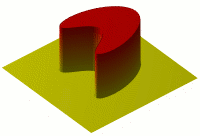or This more makes precisely, perfect sense, since the points heat equation describes how heat smears out as little particles of heat randomly move around. True, there don’t really exist ‘little particles of heat’, but the heat equation also describes the diffusion of any other kind of particles as they randomly move around undergoing Brownian motion. Similarly, our master equation describes a random walk on this segment line with segment: integer coordinates.
The equilibrium solutions arise when the probabilities $\psi_{m,n}$ have diffused as much as possible.

There’s a general moral here, too:

$m+2n = k , \qquad m , n \ge 0$

or more precisely, the points on this segment with integer coordinates. The equilibrium solutions arise when the probabilities $\psi_{m,n}$ have diffused as much as possible.

If you think about it this way, it should seem physically obvious that there’s just one linearly independent equilibrium solution of the master equation for each value of $k$.

There’s a general moral here, too, which we’re seeing in a special case: the master equation for a chemical reaction network really describes a bunch of random walks, one for each value of the conserved quantities that happen to be present. In our case we have just one conserved quantity, but in general there will be more. These ‘random walks’ are what we’ve been calling Markov processes.

### Noether's theorem

We simplified our task of finding equilibrium solutions of the master equation by finding a conserved quantity. The idea of simplifying problems using conserved quantities is fundamental to physics: this is why physicists are so enamored with quantities like energy, momentum, angular momentum and so on.

Nowadays physicists often use ‘Noether’s theorem’ to get conserved quantities from symmetries. There’s a very simple version of Noether’s theorem for quantum mechanics, but in Part 11 we saw a version for stochastic mechanics, and it’s that version that is relevant now. Here’s a paper which explains it in detail:

• John Baez and Brendan Fong, Noether’s theorem for Markov processes.

We don’t really need Noether’s theorem now, since we found the conserved quantity and exploited it without even noticing the symmetry. Nonetheless it’s interesting to see how it relates to what we’re doing.

For the reaction we’re looking at now, the idea is that the subspaces $L_k$ are eigenspaces of an operator that commutes with the Hamiltonian $H$. It follows from standard math that a solution of the master equation that starts in one of these subspaces, stays in that subspace.

What is this operator? It’s built from ‘number operators’. The <b>number operator</a> for $A$‘s is

 N_1 N_A = a_1^\dagger a^\dagger a_1 a

and the number operator for $B$‘s is

 N_2 N_B = a_2^\dagger b^\dagger a_2 b

A little calculation shows

 N_1 N_A z_1^m \,y^m z_2^n = m z_1^m \, z_2^n, y^m z^n, \quad \qquad N_1 N_B\, z_1^m y^m z_2^n z^n = m z_1^m \,y^m z_2^n z^n

so the idea is that the eigenvalue of N_1 N_A is the number of $A$‘s, while the eigenvalue of  N_2 N_B is the number of $B$ ’s. This is why they’re called number operators.

As a consequence, the eigenvalue of the operator  N_1 N_A + 2N_2 2N_B is the number of $A$‘s plus twice the number of $B$’s:

 (N_1 (N_A + 2N_2) 2N_B) z_1^m \, z_2^n y^m z^n = (m + 2n) z_1^m \, z_2^n y^m z^n

Let’s call this operator $O$, since it’s so important:

 O = N_1 + 2N_2 So, the spaces $L_k$ we saw a minute ago are just the eigenspaces of this operator: $$L_k = \{ \Psi : \; O \Psi = k \Psi \} $O = N_A + 2N_B$ As If we’ve you seen, think solutions about of it, the master spaces equation that start in one of these eigenspaces will stay there. Now, time evolution as described by the master equation is given by the operators L_k O = N_1 + 2N_2 So, the spaces . In we other saw words, a minute ago are precisely the eigenspaces of this operator:  \displaystyle{ \frac{d}{d t} \Psi(t) = H \Psi(t) \; and \;$$ \Psi(0) = \Phi \Rightarrow \Psi(t) = \exp(t H) \Phi $L_k = \{ \Psi : \; O \Psi = k \Psi \}$

So, As if we’ve seen, solutions of the master equation that start in one of these eigenspaces will stay there. This lets take some techniques that are very familiar in quantum mechanics, and apply them to this stochastic situation.$\Phi$ is an eigenvector of $O$, so is $\exp(t H) \Phi$, with the same eigenvalue. In other words,

First of all, time evolution as described by the master equation is given by the operators $\exp(t H)$. In other words,

$\displaystyle{ \frac{d}{d t} \Psi(t) } = H \Psi(t) \; and \; \Psi(0) = \Phi \quad \Rightarrow \quad \Psi(t) = \exp(t H) \Phi$

Thus if $\Phi$ is an eigenvector of $O$, so is $\exp(t H) \Phi$, with the same eigenvalue. In other words,

$O \Phi = k \Phi$

implies

$O \exp(t H) \Phi = k \exp(t H) \Phi = \exp(t H) O \Phi$

But since we can choose a basis consisting of eigenvectors of $O$, we must have

$O \exp(t H) = \exp(t H) O$

or, throwing caution to the winds and differentiating:

$O H = H O$

So, as we’d expect from Noether’s theorem, our conserved quantity commutes with the Hamiltonian! This in turn implies that $H$ commutes with any polynomial in $O$, which in turn suggests that

$\exp(s O) H = H \exp(s O)$

and then also

$\exp(s O) \exp(t H) = \exp(t H) \exp(s O)$

The last equation says that $O$ generates a 1-parameter family of ‘symmetries’ $\exp(s O)$: operators that commute with time evolution. But what do these symmetries actually do? Since

$O y^m z^n = (m + 2n) y^m z^n$

we have

$\exp(s O) y^m z^n = e^{s(m + 2n)}\, y^m z^n$

So, this symmetry takes any probability distribution $\psi_{m,n}$ and multiplies it by $e^{s(m + 2n)}$.

In other words, our symmetry multiplies the relative probability of finding our container of gas in a given state by a factor of $e^s$ for each $A$ atom, and by a factor of $e^{2s}$ for each $B$ molecule. It might not seem obvious that this operation commutes with time evolution! However, experts on chemical reaction theory are familiar with this fact.

Finally, a couple of technical points. Starting where we said ‘throwing caution to the winds’, our treatment has not been rigorous, since $O$ and $H$ are unbounded operators, and these must be handled with caution. Nonetheless, all the commutation relations we wrote down are true.

It’s also true that $\exp(s O)$ is unbounded for positive $s$. It’s bounded for negative $s$, but even then doesn’t map probability distributions to probability distributions. However, it does map any nonzero vector $\Psi$ with $\psi_{m,n} \ge 0$ to a vector $\exp(s O) \Psi$ with the same properties. So, we can just normalize this vector and get a probability distribution. This normalization is why we introduced the concept of relative probabilities.

## The Anderson-Craciun-Kurtz theorem

Here we will use the Anderson-Craciun-Kurtz theorem to work out the corresponding equilibrium states of the master equation. Brendon proved this in relation to what we consider here back in Part X.

Let

$\Psi := e^{z_1c_1 + z_2c_2}$

Then

$H\Psi = \left[ r_1(z_1^2c_2 - z_2c_2) + r_2(z_2c_2^2 - z_1^2c_1^2)\right]\Psi$

and for $\Psi \neq 0$

$(r_1 c_2 - r_2 c_1^2) z_1^2 + (r_2 c_1^2 - r_1c_2)z_2 = 0$

which vanishes for

$\frac{r_1}{r_2} = \frac{c_1^2}{c_2}$

## Noether’s theorem

Now we will show how Noether’s theorem relates the conserved quantity $2N_A + N_B$ to a symmetry.

### Conservation of particle number

The Hamiltonians that arise in Petri net field theory have a very particular general form. Not every Hamiltonian in this vast class preserves particle number (since we can have exponential growth or decay for instance). What we want to do is to find a good way to characterize those Hamiltonians that do preserve particle number. We want to understand symmetries in general. Those of you following the posts will recall the commutation relations from Part X. These are going to be relevant here too.

Just a reminder, the number operator for a single species is

$N_i = a_i^\dagger a_i$

and the number operator for all the species is a sum over the single species

$N = \sum_i N_i$

We will derive a few results at the end of the post. If you think we are telling the truth, you don’t need to check them, but they are there if you want to be bored with these sort of details. To get you into the mood…

It can be shown (using induction) that

$[a, a^\dagger^k] = k a^\dagger^{k-1}$
$[a^k,a^\dagger] = k a^{k-1}$
• exercise. Let $[a, a^\dagger] = 1$ be the base case and assume $[a, a^\dagger^k] = k a^\dagger^{k-1}$ and show that these assumptions imply the formula for $k+1$ and hence or otherwise, prove the first commutation relation listed above by induction.

Now for some results.

• (Lemma I). We arrive at the following commutation relations among number operators and general Hamiltonians in Petri net field theory
(1)$[N_i, H] = \sum_{\tau\in T} r(\tau)[n_i(\tau) - m_i(\tau)]a^\dagger^{n(\tau)}a^{m(\tau)}$

This supporting lemma can be used to prove a range of things related to symmetries in the very type of Hamiltonians we are considering here.

• (Theorem I — total particle conservation). The following quantity
(2)$\sum_{\tau\in T} r(\tau)[n(\tau) - m(\tau)]a^\dagger^{n(\tau)}a^{m(\tau)} = 0$

vanishes identically iff $H$ preserves particle number.

So to check if the total number of particles are conserved during evolution under some Hamiltonian, all one has to do is check Theorem I. The Hamiltonian we consider here does not conserve total particle number. However, the reversible reaction John did in Part 10 did.

• (Exercise). In Network Theory Part 10 John considered the reversible reaction with Hamiltonian

(3)$H = (a^\dagger - b^\dagger)(\beta b - \alpha a)$

Use Theorem I to show that this reversible reaction conserves particle number.

• (Theorem II — particle conservation symmetry). Given a Hamiltonian acting on $k$ particle species, there exists $k$ positive integer choices for $\omega_n$ which causes the following to vanish identically

(4)$\sum_i\sum_{\tau\in T} r(\tau)\omega_i[n_i(\tau) - m_i(\tau)]a^\dagger^{n(\tau)}a^{m(\tau)} = 0$

iff $H$ has a particle conservation symmetry.

As will soon be seen, this is precisely the case here. In other words, there exists $\omega_1$ and $\omega_2$ that take positive integer values which case the above quantity to vanish.

For our system to have a particle conservation symmetry, we must show that

(5)$[N_1 + 2N_2, H] = [N_1, H] + 2[N_2,H] = 0$

This vanishes since, from Lemma I, we calculate that

(6)$[N_1, H] = 2r_1 a^\dagger a^\dagger b -2 r_2 b^\dagger a a$
(7)$[N_2, H] = -r_1 a^\dagger a^\dagger b + r_2 b^\dagger a a$

Here, Theorem II applies, are we are able to find two values, $\omega_1=1$ and $\omega_2=2$ that cause the commutator to vanish. The Hamiltonian therefore has a particle number conservation symmetry.

## Master equation

Now for the master equation approach. Our Hamiltonian is given as

$H = r_1 (a^\dagger a^\dagger b - b^\dagger b) + r_2 (b^\dagger a a - a^\dagger a^\dagger a a)$

and the evolution operator at time $t$ is given as

(8)$V = e^{t H} = \sum_{k=0}^\infinity \frac{H^k}{k!}t^k$

At each order in $k$, we have a term that corresponds to the Hamiltonian $H$ acting $k$ times. One can think of these as alternative histories. In quantum mechanics, there is a spooky thing called coherence, where each of these histories seems to occur concurrently. In stochastic mechanics, each history only occurs with some probability. In terms of mathematical structure, the theories become closely related. While the semantic interpretation might differ, the syntactical form, given by a sum over histories unites quantum and stochastic mechanics. This enables us to e.g. apply tools from quantum mechanics to stochastic mechanics such as Feynman diagrams.# Supporting Material

Proof of Theorems I and II. Sometimes a long calculation can simplify matters. This is the case here, though we don’t want to muddy the water from what’s going on here, as this is just some algebra.

Here we will use the following notation.

$a^{m(\tau)}a^\dagger_i = a^{m_i(\tau)}_i a^\dagger_i a^{m'(\tau)}$
$a_i a^\dagger^{n(\tau)} = a^\dagger^{n'(\tau)}a_i a_i^\dagger^{n_i(\tau)}$

where the vector $m'(\tau)$ has its $i$th component zero which is why we are able to move term(s) $a^{m'(\tau)}$ to the right. This enables us to express the more general commutation relations,

$[a^{m(\tau)},a^\dagger_i] = m_i(\tau)a_i^{m_i(\tau)-1}a^{m'(\tau)}$
$[a_i, a^\dagger^{n(\tau)}] = n_i(\tau) (a_i^\dagger)^{n_i(\tau)-1}a^\dagger^{n'(\tau)}$

Using these relations, it follows that (is better notation possible?)

$[H, a_i^\dagger] = \sum_{\tau\in T} r(\tau)(a^\dagger^{n(\tau)} - a^\dagger^{m(\tau)})a_i^{m_i(\tau)-1} a^{m'(\tau)} m_i(\tau)$

and also

$[a_i, H] = \sum_{\tau\in T} r(\tau)[n_i(\tau)a^\dagger^{n_i(\tau)-1}a^\dagger^{n'(\tau)} - m_i(\tau)a^\dagger^{m_i(\tau)-1}a^\dagger^{m'(\tau)}]a^{m(\tau)}$

These will simplify the calculation of the commutation of the Hamiltonian and the number operator.

$[\sum_i a_i^\dagger a_i, H] = \sum_i [a_i^\dagger a_i, H] = \sum_i(a_i^\dagger [a_i, H] - [H, a_i^\dagger]a_i)$

For this to vanish, the following quantity must vanish identically.

$\sum_i (a_i^\dagger K_2 - K_1 a_i)$

where

$a_i^\dagger K_2 = a_i^\dagger[a_i, H] = \sum_i \sum_{\tau\in T} r(\tau)[n_i(\tau)a^\dagger^{n(\tau)} - m_i(\tau)a^\dagger^{m(\tau)}]a^{m(\tau)}$
$K_1 a_i = [H, a_i^\dagger]a_i = \sum_i \sum_{\tau\in T} r(\tau)(a^\dagger^{n(\tau)} - a^\dagger^{m(\tau)})a^{m(\tau)}m_i(\tau)$

Both of the theorems then follow from applications of the above.

# Solution to exercises

Particle Conservation of the simple reversible reaction. In Network Theory Part 10 John considered the reversible reaction with Hamiltonian

$H = (a^\dagger - b^\dagger)(\beta b - \alpha a)$

We find its commutation relations with the creation (destruction) operators of both species to be

$[H, a^\dagger] = \alpha (b^\dagger - a^\dagger)$
$[H, a] = \alpha a - \beta b$
$[b^\dagger, H] = \beta (b^\dagger - a^\dagger )$
$[b, H] = \alpha a - \beta b$

Now we see that the particle number is conserved for this Hamiltonian by calculating

$[N, H] = [a^\dagger a, H] + [b^\dagger b, H] = a^\dagger[a, H] + [a^\dagger, H]a + b^\dagger[b, H] + [b^\dagger, H]b = 0$

This could have also been shown, using Theorem I directly.

## New stuff

We’ve been working hard to understand the parallels and differences between quantum and stochastic mechanics. Last time we showed how the methods developed in prior posts can be used to model chemical reaction networks. This time, we are going to report the details of a battle.

This is the same quantum vs. stochastic battle we’ve talked about in prior posts, but this time we are going talk in detail about the odd nature of eigenstates in quantum mechanics, and how we can’t expect this structure in stochastic mechanics.